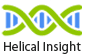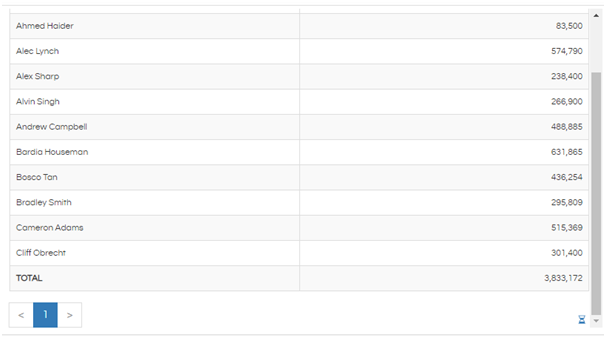# Add Total Row for Tabular Report

Hello Helical Insight,

I am using Helical Insight 4.0. In tabular report, I want to see a total row at the bottom. Is there a way to add this row?

Thank You,
Netta.

Hello Netta,

You can add a row at the bottom of the table by using the following script in the JS Editor:

``````hi_container.set("postFetch",function(c){
var responseData = c.get("responseData");
var measure1 = 0;
for(var i=0;i<responseData.data.length;i++){
measure1 += responseData.data[i].travelcost;
var fullSumMeasure1 = measure1;
}
responseData.data.push({employee_name: "<b>TOTAL</b>", travelcost: fullSumMeasure1});   c.set("responseData",responseData);
});
``````Here, you can replace the column names employee_name and travelcost with the names of the columns that you have in your report. If there are multiple columns that need to be added, define multiple measure variables (measure1, measure2 etc) and add for loop for each measure.
In case the column name has space, you can calculate the total as shown below:

``````hi_container.set("postFetch",function(c){
var responseData = c.get("responseData");
var measure1 = 0;
for(var i=0;i<responseData.data.length;i++){
measure1 += responseData.data[i]["travel cost"];
var fullSumMeasure1 = measure1;
}
responseData.data.push({employee_name: "<b>TOTAL</b>", "travel cost": fullSumMeasure1});
c.set("responseData",responseData);
});
``````

Note: The total that is calculated using the above script is calculated at the frontend. Hence, it is the total of the page that you are currently viewing. This will not display the cumulative total of all the pages if viewing the last page. Since these calculations happen at the frontend browser level it should be used only when really necessary as it can give performance issues.

Thank You,
Netta.

Note: In the settings make the data as “Full” to use this